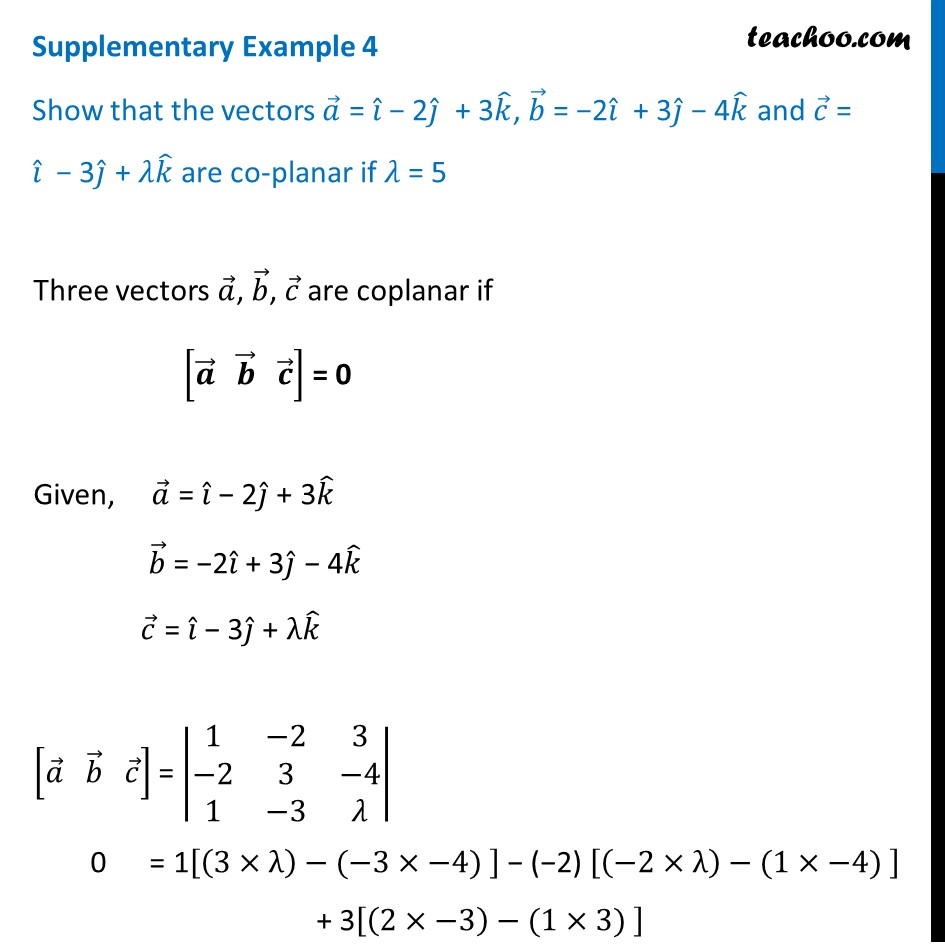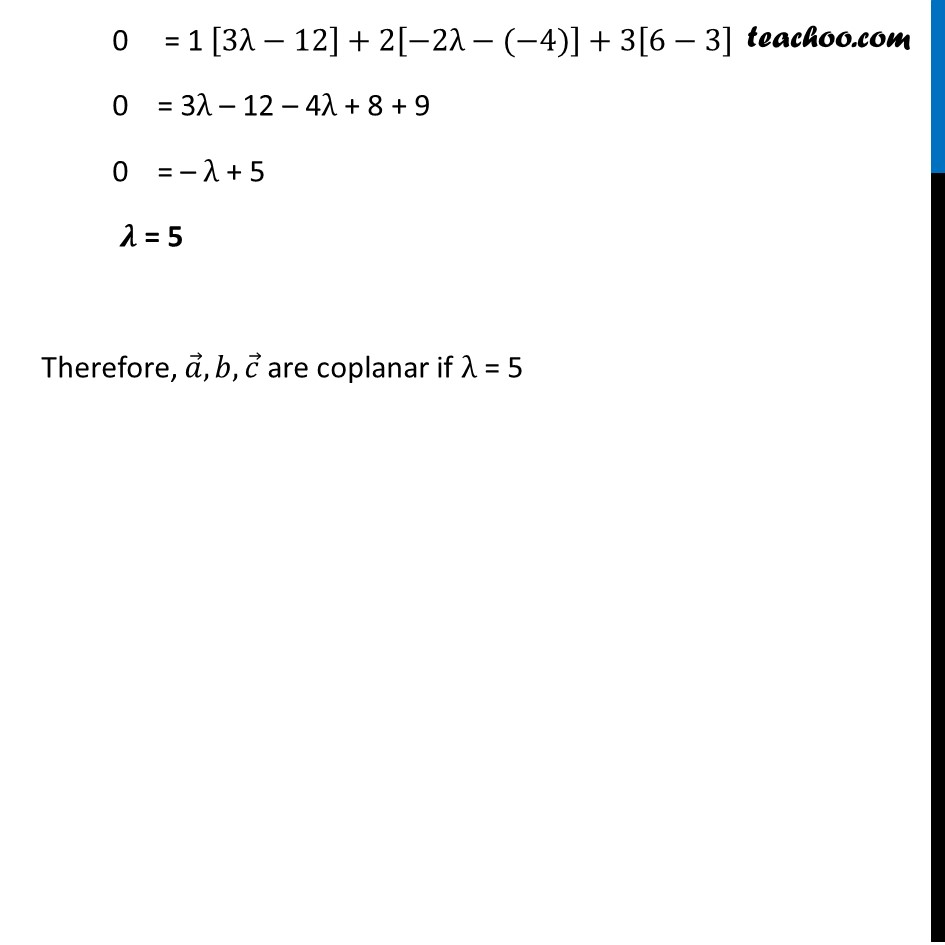Supplementary examples and questions from CBSE

Chapter 10 Class 12 Vector Algebra
Serial order wiseLearn in your speed, with individual attention - Teachoo Maths 1-on-1 Class

### Transcript

Supplementary Example 4 Show that the vectors 𝑎 ⃗ = 𝑖 ̂ − 2𝑗 ̂ + 3𝑘 ̂, 𝑏 ⃗ = −2𝑖 ̂ + 3𝑗 ̂ − 4𝑘 ̂ and 𝑐 ⃗ = 𝑖 ̂ − 3𝑗 ̂ + 𝜆𝑘 ̂ are co-planar if 𝜆 = 5 Three vectors 𝑎 ⃗, 𝑏 ⃗, 𝑐 ⃗ are coplanar if [𝒂 ⃗" " 𝒃 ⃗" " 𝒄 ⃗ ] = 0 Given, 𝑎 ⃗ = 𝑖 ̂ − 2𝑗 ̂ + 3𝑘 ̂ 𝑏 ⃗ = −2𝑖 ̂ + 3𝑗 ̂ − 4𝑘 ̂ 𝑐 ⃗ = 𝑖 ̂ − 3𝑗 ̂ + λ𝑘 ̂ [𝑎 ⃗" " 𝑏 ⃗" " 𝑐 ⃗ ] = |■8(1&−2&3@−2&3&−4@1&−3&𝜆)| 0 = 1[(3×λ)−(−3×−4) ] − (−2) [(−2×λ)−(1×−4) ] + 3[(2×−3)−(1×3) ] 0 = 1 [3λ−12]+2[−2λ−(−4)]+3[6−3] 0 = 3λ – 12 – 4λ + 8 + 9 0 = – λ + 5 𝝀 = 5 Therefore, 𝑎 ⃗,𝑏,𝑐 ⃗ are coplanar if λ = 5